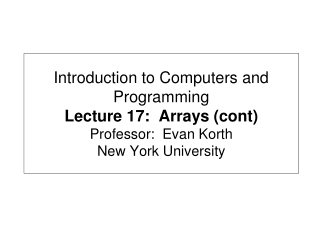DownloadDownload PresentationDownload Presentation- - - - - - - - - - - - - - - - - - - - - - - - - - - E N D - - - - - - - - - - - - - - - - - - - - - - - - - - -
##### Presentation Transcript

1. Introduction to Computers and ProgrammingLecture 17: Arrays (cont)Professor: Evan KorthNew York University

2. Road Map • More array examples. • Using methods in other files / classes • Returning arrays from methods • Copying arrays • Reading: • Liang 5: Chapter 5, Sections 5.3, 5.5 • Liang 6: Chapter 6, Sections 6.3, 6.5 • Liang 6: Chapter 6, Sections 6.3, 6.5

3. review • What is the difference between pass-by-value and pass-by-reference? • What does Java use for passing arrays? • What does Java use for primitive types? • Explain two different ways to set the size of an array in Java. • Given an array of size n, what is the valid range of subscripts? • When passing arrays in Java, when do you use the brackets? When do you omit them? (The possible answers are the method call and the method declaration) • What does MakeHot do?

4. Returning arrays from methods • We have seen examples of methods that modify an array. Since the array is passed by reference, it is modified in the original method as well. • Another way to return information in an array is to explicitly return the array. • For example, a method with the header: public static int [] makeArray () Returns an integer array.

5. Returning arrays (cont) • In order to return an array, the method creates the array first. public static int [] makeArray () { int [] myArray = new int ; for (int i; i < myArray.length; i++) array [i] = i; return myArray; } • The method above creates a new array called myArray, initializes each element and returns the reference to myArray.

6. Example 5.5 (Liang)Counting Occurrence of Each Letter • Generate 100 lowercase letters randomly and assign to an array of characters. • Count the occurrence of each letter in the array. • Find the mean and standard deviation of the counts.

7. Example 5.6 (Liang)Copying Arrays In this example, you will see that a simple assignment cannot copy arrays in the following program. The program simply creates two arrays and attempts to copy one to the other, using an assignment statement.

8. Copying Arrays (Liang)

9. Copying Arrays (Liang) Using a loop: int[] sourceArray = {2, 3, 1, 5, 10}; int[] targetArray = new int[sourceArray.length]; for (int i = 0; i < sourceArrays.length; i++) targetArray[i] = sourceArray[i];

10. The arraycopy Utility (Liang) arraycopy(sourceArray, src_pos, targetArray, tar_pos, length); Example: System.arraycopy(sourceArray, 0, targetArray, 0, sourceArray.length);